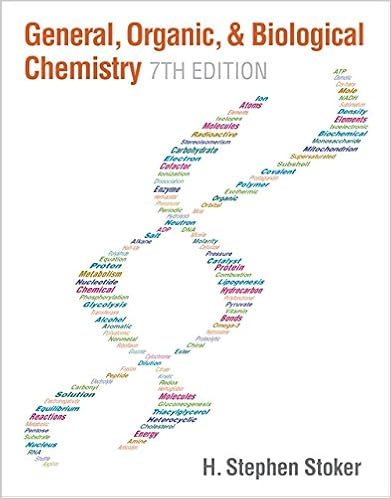# ch 4 6e - Chapter 4 Three Major Classes of Chemical...

• Notes
• 85

This preview shows page 1 - 13 out of 85 pages.

##### We have textbook solutions for you!
The document you are viewing contains questions related to this textbook.The document you are viewing contains questions related to this textbook.
Chapter 15 / Exercise 15-83
General, Organic, & Biological Chemistry
StokerExpert Verified
4-1Chapter 4Three Major Classes of Chemical Reactions
##### We have textbook solutions for you!
The document you are viewing contains questions related to this textbook.The document you are viewing contains questions related to this textbook.
Chapter 15 / Exercise 15-83
General, Organic, & Biological Chemistry
StokerExpert Verified
4-2The Major Classes of Chemical Reactions4.6 Elements in Redox Reactions4.1 The Role of Water as a Solvent4.2 Writing Equations for Aqueous Ionic Reactions4.3 Precipitation Reactions4.4 Acid-Base Reactions4.5 Oxidation-Reduction (Redox) Reactions4.7 Reaction Reversibility and the Equilibrium State
4-3
4-4Figure 4.1Electron distribution in molecules of H2and H2O.
4-5Figure 4.2An ionic compound dissolving in water.
4-6Figure 4.3The electrical conductivity of ionic solutions.
4-7Sample Problem 4.1Using Molecular Scenes to Depict an Ionic Compound in Aqueous SolutionPROBLEM:The beakers shown below contain aqueous solutions of the strong electrolyte potassium sulfate.(a)Which beaker best represents the compound in solution? (H2O molecules are not shown).(b)If each particle represents 0.10 mol, what is the total number of particles in solution?
PLAN:(a)Determine the formula and write and equation for the dissociation of 1 mol of compound. Potassium sulfate is a
(b)Count the number of separate particles in the relevant beaker, then multiply by 0.1 mol and by Avogadro’s number.Sample Problem 4.1SOLUTION:
4-9Sample Problem 4.1There should be 2 cations for every 1 anion; beaker C represents this correctly.(b) Beaker C contains 9 particles, 6 K+ions and 3 SO42-ions.= 5.420x1023particles6.022x1023particles1 mol9 x 0.1 mol x
4-10Sample Problem 4.2Determining Amount (mol) of Ions in SolutionPROBLEM:What amount (mol) of each ion is in each solution?(a)5.0 mol of ammonium sulfate dissolved in water(b)78.5 g of cesium bromide dissolved in water(c)7.42x1022formula units of copper(II) nitrate dissolved in water(d)35 mL of 0.84 Mzinc chloridePLAN:Write an equation for the dissociation of 1 mol of each compound. Use this information to calculate the actual number of moles represented by the given quantity of substance in each case.
4-11SOLUTION:= 10. mol NH4+5.0 mol (NH4)2SO4x2 mol NH4+1 mol (NH4)2SO4(a)The formula is (NH4)2SO4so the equation for dissociation is:(NH4)2SO4(s) → 2NH4+(aq) + SO42-(aq)5.0 mol (NH4)2SO4x1 mol SO42-1 mol (NH4)2SO4= 5.0 mol NH4+
Sample Problem 4.2
4-12SOLUTION:There is one Cs+ion for every Br-ion, so the number of moles of Br-is also equation to 0.369 mol.= 0.369 mol Cs+78.5 g CsBr x1 mol CsBr 212.8 g CsBr 1 mol Cs+1 mol CsBrx(b)The formula is CsBr so the equation for dissociation is:CsBr (s) → Cs+(aq) + Br-(aq)Sample Problem 4.2
•••